xOrbital hybridisationOverview

In chemistry
Chemistry
Chemistry is the science of matter, especially its chemical reactions, but also its composition, structure and properties. Chemistry is concerned with atoms and their interactions with other atoms, and particularly with the properties of chemical bonds....

, hybridisation (or hybridization) is the concept of mixing atomic orbital
Atomic orbital
An atomic orbital is a mathematical function that describes the wave-like behavior of either one electron or a pair of electrons in an atom. This function can be used to calculate the probability of finding any electron of an atom in any specific region around the atom's nucleus...

s to form new hybrid orbitals suitable for the qualitative description of atomic bonding properties. Hybridised orbitals are very useful in the explanation of the shape of molecular orbital
Molecular orbital
In chemistry, a molecular orbital is a mathematical function describing the wave-like behavior of an electron in a molecule. This function can be used to calculate chemical and physical properties such as the probability of finding an electron in any specific region. The term "orbital" was first...

s for molecule
Molecule
A molecule is an electrically neutral group of at least two atoms held together by covalent chemical bonds. Molecules are distinguished from ions by their electrical charge...

s. It is an integral part of valence bond theory
Valence bond theory
In chemistry, valence bond theory is one of two basic theories, along with molecular orbital theory, that were developed to use the methods of quantum mechanics to explain chemical bonding. It focuses on how the atomic orbitals of the dissociated atoms combine to give individual chemical bonds...

. Although sometimes taught together with the valence shell electron-pair repulsion (VSEPR) theory, valence bond and hybridization are in fact not related to the VSEPR model.
Chemist
Chemist
A chemist is a scientist trained in the study of chemistry. Chemists study the composition of matter and its properties such as density and acidity. Chemists carefully describe the properties they study in terms of quantities, with detail on the level of molecules and their component atoms...

Linus Pauling
Linus Pauling
Linus Carl Pauling was an American chemist, biochemist, peace activist, author, and educator. He was one of the most influential chemists in history and ranks among the most important scientists of the 20th century...

first developed the hybridisation theory in order to explain the structure of molecule
Molecule
A molecule is an electrically neutral group of at least two atoms held together by covalent chemical bonds. Molecules are distinguished from ions by their electrical charge...

s such as methane
Methane
Methane is a chemical compound with the chemical formula . It is the simplest alkane, the principal component of natural gas, and probably the most abundant organic compound on earth. The relative abundance of methane makes it an attractive fuel...

(CH4).How to explain the formation of OCl2 using hybridisation theory ?What is the hybridisation in phosphenewhat is the hybridization of phosphorous in phosphine?Encyclopedia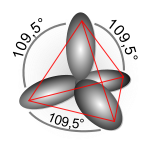In chemistry
Chemistry
Chemistry is the science of matter, especially its chemical reactions, but also its composition, structure and properties. Chemistry is concerned with atoms and their interactions with other atoms, and particularly with the properties of chemical bonds....

, hybridisation (or hybridization) is the concept of mixing atomic orbital
Atomic orbital
An atomic orbital is a mathematical function that describes the wave-like behavior of either one electron or a pair of electrons in an atom. This function can be used to calculate the probability of finding any electron of an atom in any specific region around the atom's nucleus...

s to form new hybrid orbitals suitable for the qualitative description of atomic bonding properties. Hybridised orbitals are very useful in the explanation of the shape of molecular orbital
Molecular orbital
In chemistry, a molecular orbital is a mathematical function describing the wave-like behavior of an electron in a molecule. This function can be used to calculate chemical and physical properties such as the probability of finding an electron in any specific region. The term "orbital" was first...

s for molecule
Molecule
A molecule is an electrically neutral group of at least two atoms held together by covalent chemical bonds. Molecules are distinguished from ions by their electrical charge...

s. It is an integral part of valence bond theory
Valence bond theory
In chemistry, valence bond theory is one of two basic theories, along with molecular orbital theory, that were developed to use the methods of quantum mechanics to explain chemical bonding. It focuses on how the atomic orbitals of the dissociated atoms combine to give individual chemical bonds...

. Although sometimes taught together with the valence shell electron-pair repulsion (VSEPR) theory, valence bond and hybridization are in fact not related to the VSEPR model.

## Historical development

Chemist
Chemist
A chemist is a scientist trained in the study of chemistry. Chemists study the composition of matter and its properties such as density and acidity. Chemists carefully describe the properties they study in terms of quantities, with detail on the level of molecules and their component atoms...

Linus Pauling
Linus Pauling
Linus Carl Pauling was an American chemist, biochemist, peace activist, author, and educator. He was one of the most influential chemists in history and ranks among the most important scientists of the 20th century...

first developed the hybridisation theory in order to explain the structure of molecule
Molecule
A molecule is an electrically neutral group of at least two atoms held together by covalent chemical bonds. Molecules are distinguished from ions by their electrical charge...

s such as methane
Methane
Methane is a chemical compound with the chemical formula . It is the simplest alkane, the principal component of natural gas, and probably the most abundant organic compound on earth. The relative abundance of methane makes it an attractive fuel...

(CH4). This concept was developed for such simple chemical systems, but the approach was later applied more widely, and today it is considered an effective heuristic for rationalizing the structures of organic compounds.

Hybridisation theory is not as practical for quantitative calculations as molecular orbital theory
Molecular orbital theory
In chemistry, molecular orbital theory is a method for determining molecular structure in which electrons are not assigned to individual bonds between atoms, but are treated as moving under the influence of the nuclei in the whole molecule...

. Problems with hybridisation are especially notable when the d orbitals are involved in bonding, as in coordination chemistry and organometallic chemistry
Organometallic chemistry
Organometallic chemistry is the study of chemical compounds containing bonds between carbon and a metal. Since many compounds without such bonds are chemically similar, an alternative may be compounds containing metal-element bonds of a largely covalent character...

. Although hybridisation schemes in transition metal chemistry can be used, they are not generally as accurate.

Orbitals are a model representation of the behaviour of electrons within molecules. In the case of simple hybridisation, this approximation is based on atomic orbitals, similar to those obtained for the hydrogen atom, the only atom for which an exact analytic solution to its Schrödinger equation
Schrödinger equation
The Schrödinger equation was formulated in 1926 by Austrian physicist Erwin Schrödinger. Used in physics , it is an equation that describes how the quantum state of a physical system changes in time....

is known. In heavier atoms, like carbon, nitrogen, and oxygen, the atomic orbitals used are the 2s and 2p orbitals, similar to excited state orbitals for hydrogen. Hybridised orbitals are assumed to be mixtures of these atomic orbitals, superimposed on each other in various proportions. The theory of hybridisation is most applicable under these assumptions. It gives a simple orbital picture equivalent to Lewis structure
Lewis structure
Lewis structures are diagrams that show the bonding between atoms of a molecule and the lone pairs of electrons that may exist in the molecule. A Lewis structure can be drawn for any covalently bonded molecule, as well as coordination compounds...

s. Hybridisation is not required to describe molecules, but for molecules made up from carbon
Carbon
Carbon is the chemical element with symbol C and atomic number 6. As a member of group 14 on the periodic table, it is nonmetallic and tetravalent—making four electrons available to form covalent chemical bonds...

, nitrogen
Nitrogen
Nitrogen is a chemical element that has the symbol N, atomic number of 7 and atomic mass 14.00674 u. Elemental nitrogen is a colorless, odorless, tasteless, and mostly inert diatomic gas at standard conditions, constituting 78.08% by volume of Earth's atmosphere...

and oxygen
Oxygen
Oxygen is the element with atomic number 8 and represented by the symbol O. Its name derives from the Greek roots ὀξύς and -γενής , because at the time of naming, it was mistakenly thought that all acids required oxygen in their composition...

(and to a lesser extent, sulfur
Sulfur
Sulfur or sulphur is the chemical element with atomic number 16. In the periodic table it is represented by the symbol S. It is an abundant, multivalent non-metal. Under normal conditions, sulfur atoms form cyclic octatomic molecules with chemical formula S8. Elemental sulfur is a bright yellow...

and phosphorus
Phosphorus
Phosphorus is the chemical element that has the symbol P and atomic number 15. A multivalent nonmetal of the nitrogen group, phosphorus as a mineral is almost always present in its maximally oxidized state, as inorganic phosphate rocks...

) the hybridisation theory/model makes the description much easier.

The hybridisation theory finds its use mainly in organic chemistry. Its explanation starts with the way bonding is organized in methane
Methane
Methane is a chemical compound with the chemical formula . It is the simplest alkane, the principal component of natural gas, and probably the most abundant organic compound on earth. The relative abundance of methane makes it an attractive fuel...

.

### sp3 hybrids

Hybridisation describes the bonding atoms from an atom's point of view. That is, for a tetrahedrally coordinated carbon (e.g., methane
Methane
Methane is a chemical compound with the chemical formula . It is the simplest alkane, the principal component of natural gas, and probably the most abundant organic compound on earth. The relative abundance of methane makes it an attractive fuel...

, CH4), the carbon should have 4 orbitals with the correct symmetry to bond to the 4 hydrogen atoms. The problem with the existence of methane is now this: carbon's ground state
Ground state
The ground state of a quantum mechanical system is its lowest-energy state; the energy of the ground state is known as the zero-point energy of the system. An excited state is any state with energy greater than the ground state...

configuration is 1s2 2s2 2px1 2py1 or more easily read: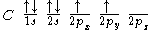The valence bond theory
Valence bond theory
In chemistry, valence bond theory is one of two basic theories, along with molecular orbital theory, that were developed to use the methods of quantum mechanics to explain chemical bonding. It focuses on how the atomic orbitals of the dissociated atoms combine to give individual chemical bonds...

would predict, based on the existence of two half-filled p-type orbitals (the designations px py or pz are meaningless at this point, as they do not fill in any particular order), that C forms two covalent bond
Covalent bond
A covalent bond is a form of chemical bonding that is characterized by the sharing of pairs of electrons between atoms. The stable balance of attractive and repulsive forces between atoms when they share electrons is known as covalent bonding....

s, i.e., CH2 (methylene
Methylene
Methylene is a chemical species in which a carbon atom is bonded to two hydrogen atoms. Three different possibilities present themselves:* the -CH2- substituent group: e.g., dichloromethane ....

Carbene
In chemistry, a carbene is a molecule containing a neutral carbon atom with a valence of two and two unshared valence electrons. The general formula is RR'C:, but the carbon can instead be double-bonded to one group. The term "carbene" may also merely refer to the compound H2C:, also called...

) and cannot exist outside of a molecular system. Therefore, this theory alone cannot explain the existence of CH4.

Furthermore, ground state orbitals cannot be used for bonding in CH4. While exciting a 2s electron into a 2p orbital would, in theory, allow for four bonds according to the valence bond theory, (which has been proved experimentally correct for systems like O2) this would imply that the various bonds of CH4 would have differing energies due to differing levels of orbital overlap
Orbital overlap
Orbital overlap was an idea first introduced by Linus Pauling to explain the molecular bond angles observed through experimentation and is the basis for the concept of orbital hybridisation. s orbitals are spherical and have no directionality while p orbitals are oriented 90° to one another...

. Once again, this has been experimentally disproved: any hydrogen can be removed from a carbon with equal ease.

To summarise, to explain the existence of CH4 (and many other molecules) a method by which as many as 12 bonds (for transition metal
Transition metal
The term transition metal has two possible meanings:*The IUPAC definition states that a transition metal is "an element whose atom has an incomplete d sub-shell, or which can give rise to cations with an incomplete d sub-shell." Group 12 elements are not transition metals in this definition.*Some...

s) of equal strength (and therefore equal length) was required.

The first step in hybridisation is the excitation of one (or more) electrons (we consider the carbon atom in methane, for simplicity of the discussion):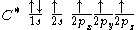The proton that forms the nucleus of a hydrogen atom attracts one of the lower-energy valence electrons on carbon. This causes an excitation, moving a 2s electron into a 2p orbital. This, however, increases the influence of the carbon nucleus on the valence electrons by increasing the effective core potential (the amount of charge the nucleus exerts on a given electron = Charge of Core − Charge of all electrons closer to the nucleus). The effective core potential is also known as the effective nuclear charge, or Zeff.

The solution to the Schrödinger equation for this configuration is a linear combination of the s and p wave functions, or orbitals, known as a hybridized orbital. In the case of carbon attempting to bond with four hydrogens, four orbitals are required. Therefore, the 2s orbital (core orbitals are almost never involved in bonding) "mixes" with the three 2p orbitals to form four sp3 hybrids (read as s-p-three). See graphical summary below.

becomes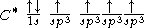In CH4, four sp3 hybridised orbitals are overlapped by hydrogen
Hydrogen
Hydrogen is the chemical element with atomic number 1. It is represented by the symbol H. With an average atomic weight of , hydrogen is the lightest and most abundant chemical element, constituting roughly 75% of the Universe's chemical elemental mass. Stars in the main sequence are mainly...

's 1s orbital, yielding four σ (sigma) bonds
Sigma bond
In chemistry, sigma bonds are the strongest type of covalent chemical bond. They are formed by head-on overlapping between atomic orbitals. Sigma bonding is most clearly defined for diatomic molecules using the language and tools of symmetry groups. In this formal approach, a σ-bond is...

(that is, four single covalent bonds). The four bonds are of the same length and strength. This theory fits our requirements.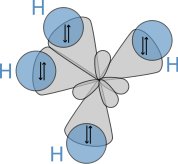translates into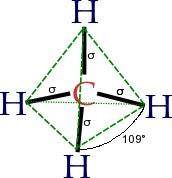An alternative view is: View the carbon as the C4− anion. In this case all the orbitals on the carbon are filled: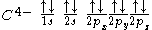If we now recombine these orbitals with the empty s-orbitals of 4 hydrogens (4 protons, H+) and then allow maximum separation between the 4 hydrogens (i.e., tetrahedral surrounding of the carbon), we see that at any orientation of the p-orbitals, a single hydrogen has an overlap of 25% with the s-orbital of the C, and a total of 75% of overlap with the 3 p-orbitals (see that the relative percentages are the same as the character of the respective orbital in an sp3-hybridisation model, 25% s- and 75% p-character).

According to the orbital hybridisation theory, the valence electrons in methane should be equal in energy but its photoelectron spectrum shows two bands, one at 12.7 eV (one electron pair) and one at 23 eV (three electron pairs). This apparent inconsistency can be explained when one considers additional orbital mixing taking place when the sp3 orbitals mix with the 4 hydrogen orbitals.

### sp2 hybridsOther carbon based compounds and other molecules may be explained in a similar way as methane. Take, for example, ethene (C2H4). Ethene has a double bond between the carbons.

For this molecule, carbon will sp2 hybridise, because one π (pi) bond
Pi bond
In chemistry, pi bonds are covalent chemical bonds where two lobes of one involved atomic orbital overlap two lobes of the other involved atomic orbital...

is required for the double bond
Covalent bond
A covalent bond is a form of chemical bonding that is characterized by the sharing of pairs of electrons between atoms. The stable balance of attractive and repulsive forces between atoms when they share electrons is known as covalent bonding....

between the carbons, and only three σ bonds are formed per carbon atom. In sp2 hybridisation the 2s orbital is mixed with only two of the three available 2p orbitals: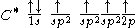forming a total of 3 sp2 orbitals with one p-orbital remaining. In ethylene (ethene) the two carbon atoms form a σ bond by overlapping two sp2 orbitals and each carbon atom forms two covalent bonds with hydrogen by s–sp2 overlap all with 120° angles. The π bond between the carbon atoms perpendicular to the molecular plane is formed by 2p–2p overlap. The hydrogen–carbon bonds are all of equal strength and length, which agrees with experimental data.

The amount of p-character is not restricted to integer values; i.e., hybridisations like sp2.5 are also readily described. In this case the geometries are somewhat distorted from the ideally hybridised picture. For example, as stated in Bent's rule
Bent's rule
Bent’s rule was formulated in 1961 by American chemist, Henry Bent, to explain deviations in structures predicted from the VSEPR theory. The rule states: “atomic s character tends to concentrate in orbitals that are directed toward electropositive groups and atomic p character tends to concentrate...

, a bond tends to have higher p-character when directed toward a more electronegative
Electronegativity
Electronegativity, symbol χ , is a chemical property that describes the tendency of an atom or a functional group to attract electrons towards itself. An atom's electronegativity is affected by both its atomic number and the distance that its valence electrons reside from the charged nucleus...

substituent.

### sp hybrids

The chemical bonding in compounds such as alkyne
Alkyne
Alkynes are hydrocarbons that have a triple bond between two carbon atoms, with the formula CnH2n-2. Alkynes are traditionally known as acetylenes, although the name acetylene also refers specifically to C2H2, known formally as ethyne using IUPAC nomenclature...

s with triple bonds is explained by sp hybridization.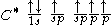In this model, the 2s orbital mixes with only one of the three p-orbitals resulting in two sp orbitals and two remaining unchanged p orbitals. The chemical bonding in acetylene
Acetylene
Acetylene is the chemical compound with the formula C2H2. It is a hydrocarbon and the simplest alkyne. This colorless gas is widely used as a fuel and a chemical building block. It is unstable in pure form and thus is usually handled as a solution.As an alkyne, acetylene is unsaturated because...

(ethyne) (C2H2) consists of sp–sp overlap between the two carbon atoms forming a σ bond and two additional π bonds formed by p–p overlap. Each carbon also bonds to hydrogen in a sigma s–sp overlap at 180° angles.

## Hybridisation and molecule shape

Hybridisation, along with the VSEPR theory
VSEPR theory
Valence shell electron pair repulsion theory is a model in chemistry used to predict the shape of individual molecules based upon the extent of electron-pair electrostatic repulsion. It is also named Gillespie–Nyholm theory after its two main developers...

, helps to explain molecule shape:
• AX1 (e.g., LiH): no hybridisation; trivially linear shape
• AX2 (e.g., BeCl2): sp hybridisation; linear
Line (mathematics)
The notion of line or straight line was introduced by the ancient mathematicians to represent straight objects with negligible width and depth. Lines are an idealization of such objects...

or digonal shape; bond angles are cos−1(−1) = 180°
• AX3 (e.g., BCl3): sp2 hybridisation; trigonal planar
Trigonal planar
In chemistry, trigonal planar is a molecular geometry model with one atom at the center and three atoms at the corners of a triangle, called peripheral atoms, all in one plane. In an ideal trigonal planar species, all three ligands are identical and all bond angles are 120°. Such species belong to...

shape; bond angles are cos−1(−1/2) = 120°
• AX2E (e.g., GeF2): bent / V shape, < 120°
• AX4 (e.g., CCl4): sp3 hybridisation; tetrahedral
Tetrahedral molecular geometry
In a tetrahedral molecular geometry a central atom is located at the center with four substituents that are located at the corners of a tetrahedron. The bond angles are cos−1 ≈ 109.5° when all four substituents are the same, as in CH4. This molecular geometry is common throughout the first...

shape; bond angles are cos−1(−1/3) ≈ 109.5°
• AX3E (e.g.,NH3): trigonal pyramidal, 107° (Note: The existence of a lone pair of electrons distorts bond angles slightly due to increased s-orbital character in the lone pair and increased p-orbital character in the orbitals used to make the bond pairs. It is not due to increased electron repulsion which is a very common misconception.)
• AX5 (e.g., PCl5): sp3d hybridisation; trigonal bipyramidal
Trigonal bipyramid molecular geometry
In chemistry a trigonal bipyramid formation is a molecular geometry with one atom at the center and 5 more atoms at the corners of a triangular dipyramid...

shape
• AX6 (e.g., SF6): sp3d2 hybridisation; octahedral
Octahedral molecular geometry
In chemistry, octahedral molecular geometry describes the shape of compounds where in six atoms or groups of atoms or ligands are symmetrically arranged around a central atom, defining the vertices of an octahedron...

(or square bipyramidal) shape

This holds if there are no lone electron pairs on the central atom. If there are, they should be counted in the Xi number, but bond angles become smaller due to increased repulsion. For example, in water
Water
Water is a chemical substance with the chemical formula H2O. A water molecule contains one oxygen and two hydrogen atoms connected by covalent bonds. Water is a liquid at ambient conditions, but it often co-exists on Earth with its solid state, ice, and gaseous state . Water also exists in a...

(H2O), the oxygen
Oxygen
Oxygen is the element with atomic number 8 and represented by the symbol O. Its name derives from the Greek roots ὀξύς and -γενής , because at the time of naming, it was mistakenly thought that all acids required oxygen in their composition...

atom has two bonds with H and two lone electron pairs (as can be seen with the valence bond theory as well from the electronic configuration of oxygen), which means there are four such 'elements' on O. The model molecule is, then, AX2E2: sp3 hybridisation is utilized, and the electron arrangement of H2O is tetrahedral. This agrees with the experimentally-determined shape for water, a non-linear, bent structure, with a bond angle of 104.5 degrees (the two lone-pairs are not visible).

In general, for an atom with s and p orbitals forming hybrids hi and hj with included angle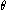, the following holds: 1 +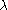ij cos() = 0. The p-to-s ratio for hybrid i isi2, and for hybrid j it isj2. In the special case of equivalent hybrids on the same atom, again with included angle, the equation reduces to just 1 +2 cos() = 0. For example, BH3 has a trigonal planar geometry, three 120o bond angles, three equivalent hybrids about the boron atom, and thus 1 +2 cos() = 0 becomes 1 +2 cos(120o) = 0, giving2 = 2 for the p-to-s ratio. In other words, sp2 hybrids, just as expected from the list above.

### Explanation of the shape of water

Commonly, the hybridization of the oxygen in water is described as sp3 following the guidelines of VSEPR and the tetrahedral electron geometry it implies. In order for this to be true, the two electron pairs would be in equal-energy, symmetrical, sp3 hybridised orbitals (two electron-pairs and two hydrogen atoms making the tetrahedron). However, molecular orbital
Molecular orbital
In chemistry, a molecular orbital is a mathematical function describing the wave-like behavior of an electron in a molecule. This function can be used to calculate chemical and physical properties such as the probability of finding an electron in any specific region. The term "orbital" was first...

s calculations give orbitals which reflect the symmetry of the molecule. One of the two lone pairs is in a pure p-type orbital, with its electron density perpendicular to the H-O-H framework. The other lone pair is an orbital that is close to an sp2-type orbital that is in the same plane as the H-O-H bonding. It is not a purely sp2-type orbital, but is extra rich in s-character. Photoelectron spectra
Ultraviolet photoelectron spectroscopy
Ultraviolet photoelectron spectroscopy refers to the measurement of kinetic energy spectra of photoelectrons emitted by molecules which have absorbed ultraviolet photons, in order to determine molecular energy levels in the valence region.-Basic Theory:...

confirm the presence of two different energies for the nonbonded electrons.

In contrast, the orbitals used to make the O-H bonds are close to sp2 hybrids, but are extra p-rich. However, molecular orbital theory does not give two equivalent bonds, but two delocalised orbitals which are in-phase and out-of-phase combinations of the H-O bond orbitals. It has been argued that it is this change in the mixing of the orbitals that is responsible for the compression of the H-O-H angle down to the experimental 104.5 degrees, not some change in the repulsion of electrons. However, if the molecular orbitals are localised
Localized molecular orbitals
Localized molecular orbitals are molecular orbitals which are concentrated in a limited spatial region of a molecule, for example a specific bond or a lone pair on a specific atom...

, leaving the total wave function unaltered, one obtains two equivalent lone pairs and two equivalent bonds.

An accurate prediction of the bond angle requires however that polarisation d functions be added to the molecular orbital calculation. Thus while VSEPR and its application to hybridisation predicts the correct atomic framework for water, it may do so for the wrong reason.

### Controversy regarding d-orbital participation

Hybridisation theory has failed in a few aspects, notably in explaining the energy considerations for the involvement of d-orbitals in chemical bonding (See above for sp3d and sp3d2 hybridisation). This can be well-explained by means of an example. Consider, for instance, how the theory in question accounts for the bonding in phosphorus pentachloride (PCl5). The d-orbitals are large, comparatively distant from the nucleus and high in energy. Radial distances of orbitals from the nucleus seem to reveal that d-orbitals are far too high in energy to 'mix' with s- and p-orbitals. 3s – 0.47 , 3p – 0.55, 3d – 2.4 (in angstrom
Ångström
The angstrom or ångström, is a unit of length equal to 1/10,000,000,000 of a meter . Its symbol is the Swedish letter Å....

s). Thus, at first glance, sp3d hybridisation seems improbable.

However, a closer examination of the factors that affect orbital size (and energy) reveals more. Formal charge
Formal charge
In chemistry, a formal charge is the charge assigned to an atom in a molecule, assuming that electrons in a chemical bond are shared equally between atoms, regardless of relative electronegativity....

on the central atom is one such factor, and it is obvious that the P atom in PCl5 carries quite a large partial positive charge. Thus the 3d orbital contracts in size to such an extent that hybridisation with s and p orbitals may occur. Further, note the cases in which d-orbital participation was proposed in hybridisation: SF6(sulfur hexafluoride
Sulfur hexafluoride
Sulfur hexafluoride is an inorganic, colorless, odorless, and non-flammable greenhouse gas. has an octahedral geometry, consisting of six fluorine atoms attached to a central sulfur atom. It is a hypervalent molecule. Typical for a nonpolar gas, it is poorly soluble in water but soluble in...

), IF7, XeF6; in all these molecules, the central atom is surrounded by the highly electronegative fluorine
Fluorine
Fluorine is the chemical element with atomic number 9, represented by the symbol F. It is the lightest element of the halogen column of the periodic table and has a single stable isotope, fluorine-19. At standard pressure and temperature, fluorine is a pale yellow gas composed of diatomic...

atom, thus making hybridisation probable among s, p and d orbitals. A further study reveals that orbital size also depends on the number of electrons occupying it. And, even further, coupling of d orbital electrons also results in contraction, albeit to a lesser extent.

The molecular orbital theory
Molecular orbital theory
In chemistry, molecular orbital theory is a method for determining molecular structure in which electrons are not assigned to individual bonds between atoms, but are treated as moving under the influence of the nuclei in the whole molecule...

, however, offers a clearer insight into the bonding in these molecules.

## Hybridisation theory vs. MO theory

Hybridisation theory is an integral part of organic chemistry
Organic chemistry
Organic chemistry is a subdiscipline within chemistry involving the scientific study of the structure, properties, composition, reactions, and preparation of carbon-based compounds, hydrocarbons, and their derivatives...

and in general discussed together with molecular orbital theory
Molecular orbital theory
In chemistry, molecular orbital theory is a method for determining molecular structure in which electrons are not assigned to individual bonds between atoms, but are treated as moving under the influence of the nuclei in the whole molecule...

in advanced organic chemistry textbooks although for different reasons. One textbook notes that for drawing reaction mechanisms sometimes a classical bonding picture is needed with two atoms sharing two electrons. It also comments that predicting bond angles in methane with MO theory is not straightforward. Another textbook treats hybridisation theory when explaining bonding in alkenes and a third uses MO theory to explain bonding in hydrogen but hybridisation theory for methane.

Although the language and pictures arising from hybridisation theory, more widely known as valence bond theory
Valence bond theory
In chemistry, valence bond theory is one of two basic theories, along with molecular orbital theory, that were developed to use the methods of quantum mechanics to explain chemical bonding. It focuses on how the atomic orbitals of the dissociated atoms combine to give individual chemical bonds...

, remain widespread in synthetic organic chemistry
Organic synthesis
Organic synthesis is a special branch of chemical synthesis and is concerned with the construction of organic compounds via organic reactions. Organic molecules can often contain a higher level of complexity compared to purely inorganic compounds, so the synthesis of organic compounds has...

, this qualitative view of bonding has been largely superseded by molecular orbital theory when a more detailed analysis is required. Advanced texts often stress that while hybrid orbital theory is still useful for problems requiring a rough approximation, it provides an incomplete picture that cannot account for many chemical phenomena. One specific problem with hybridisation is that it incorrectly predicts the photoelectron spectra of many molecules, including such fundamental species such as methane and water. From a pedagogical perspective, hybridisation approach tends to over-emphasize localisation of bonding electrons and does not effectively embrace molecular symmetry
Molecular symmetry
Molecular symmetry in chemistry describes the symmetry present in molecules and the classification of molecules according to their symmetry. Molecular symmetry is a fundamental concept in chemistry, as it can predict or explain many of a molecule's chemical properties, such as its dipole moment...

as does MO theory.

Bonding orbitals formed from hybrid atomic orbitals may be considered as localized molecular orbitals
Localized molecular orbitals
Localized molecular orbitals are molecular orbitals which are concentrated in a limited spatial region of a molecule, for example a specific bond or a lone pair on a specific atom...

, which can be formed from the delocalized orbitals of molecular orbital theory by an appropriate mathematical transformation. For molecules with a closed electron shell in the ground state, this transformation of the orbitals leaves the total many-electron wave function unchanged. The hybrid orbital description of the ground state is therefore equivalent to the delocalized orbital description for explaining the ground state total energy and electron density, as well as the molecular geometry which corresponds to the minimum value of the total energy.

There is no such equivalence, however, for ionized or excited states with open electron shells. Hybrid orbitals cannot therefore be used to interpret photoelectron spectra, which measure the energies of ionized states, identified with delocalized orbital energies using Koopmans' theorem
Koopmans' theorem
Koopmans' theorem states that in closed-shell Hartree-Fock theory, the first ionization energy of a molecular system is equal to the negative of the orbital energy of the highest occupied molecular orbital...

. Nor can they be used to interpret UV-visible spectra which correspond to electronic transitions between delocalized orbitals.

#### There are six steps to identify the hybrid state:

1. Locate the central element.
2. Identify the valence electrons of the central element.
3. If there is a positive charge, subtract it from the valency of the central element
4. If there is a negative charge, add it to the valency of the central element.
5. Neglect oxygen & it's family(don't neglect its charge)and add number of other elements to the valance electrons of the central element.
6. Dividing by two gives the number of hybrid orbitals.

#### Exceptions.

1. If oxygen is the central element (e.g- H2O,OCl2),apply the steps on the central oxygen atom.
2. Doesn't apply on multiple central element organic compounds and multiple central element compounds.(can be identified by the structure)
3. Doesn't apply on the following elements such as NO(sp), ClO2(sp²), NO2(sp²), O3, S8 & P4. (please post if any other exceptions are present)

• Linear combination of atomic orbitals molecular orbital method
Linear combination of atomic orbitals molecular orbital method
A linear combination of atomic orbitals or LCAO is a quantum superposition of atomic orbitals and a technique for calculating molecular orbitals in quantum chemistry. In quantum mechanics, electron configurations of atoms are described as wavefunctions...

• MO diagram
MO diagram
A molecular orbital diagram, or MO diagram for short, is a qualitative descriptive tool explaining chemical bonding in molecules in terms of molecular orbital theory in general and the Linear combination of atomic orbitals molecular orbital method in particular...

s